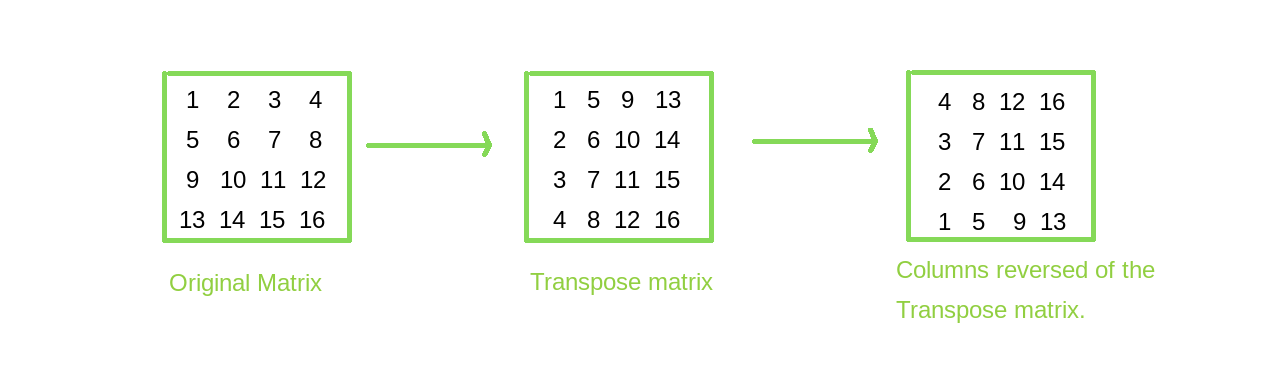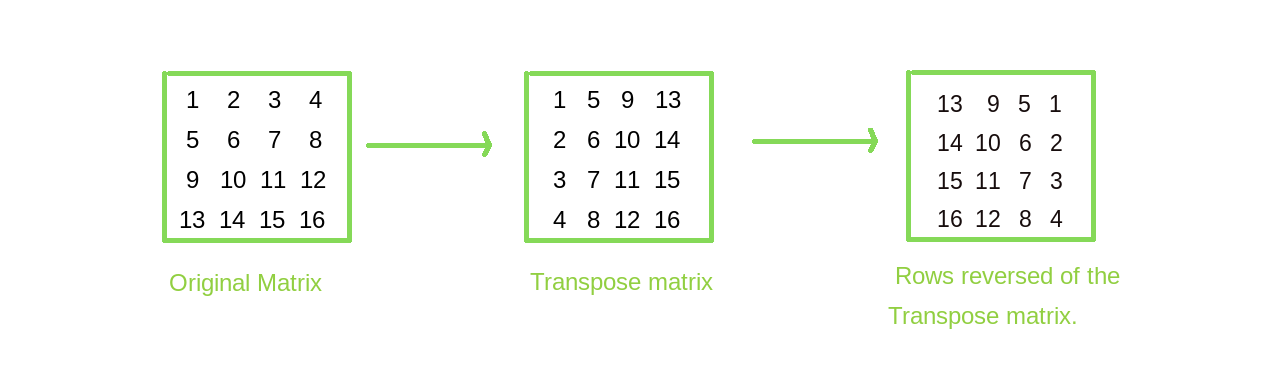# Rotate a matrix by 90 degree without using any extra space | Set 2

Given a square matrix, turn it by 90 degrees in anti-clockwise direction without using any extra space.

Examples:

```Input:
1  2  3
4  5  6
7  8  9
Output:
3  6  9
2  5  8
1  4  7
Rotated the input matrix by
90 degrees in anti-clockwise direction.

Input:
1  2  3  4
5  6  7  8
9 10 11 12
13 14 15 16
Output:
4  8 12 16
3  7 11 15
2  6 10 14
1  5  9 13
Rotated the input matrix by
90 degrees in anti-clockwise direction.
```

## Recommended: Please try your approach on {IDE} first, before moving on to the solution.

An approach that requires extra space is already discussed in a different article:
Inplace rotate square matrix by 90 degrees | Set 1

This post discusses the same problem with a different approach which is space-optimized.

Approach: The idea is to find the transpose of the matrix and then reverse the columns of the transposed matrix.
Here is an example to show how this works.Algorithm:

1. To solve the given problem there are two tasks. 1st is finding the transpose and second is reversing the columns without using extra space
2. A transpose of a matrix is when the matrix is flipped over its diagonal, i.e the row index of an element becomes the column index and vice versa. So to find the transpose interchange the elements at position (i, j) with (j, i). Run two loops, the outer loop from 0 to row count and inner loop from 0 to index of the outer loop.
3. To reverse the column of the transposed matrix, run two nested loops, the outer loop from 0 to column count and inner loop from 0 to row count/2, interchange elements at (i, j) with (i, row[count-1-j]), where i and j are indices of inner and outer loop respectively.

## C++

 `// C++ program for left ` `// rotation of matrix by 90 degree ` `// without using extra space ` `#include ` `using` `namespace` `std; ` `#define R 4 ` `#define C 4 ` ` `  `// After transpose we swap ` `// elements of column ` `// one by one for finding left ` `// rotation of matrix ` `// by 90 degree ` `void` `reverseColumns(``int` `arr[R][C]) ` `{ ` `    ``for` `(``int` `i = 0; i < C; i++) ` `        ``for` `(``int` `j = 0, k = C - 1; ` `             ``j < k; j++, k--) ` `            ``swap( ` `                ``arr[j][i], arr[k][i]); ` `} ` ` `  `// Function for do transpose of matrix ` `void` `transpose(``int` `arr[R][C]) ` `{ ` `    ``for` `(``int` `i = 0; i < R; i++) ` `        ``for` `(``int` `j = i; j < C; j++) ` `            ``swap(arr[i][j], arr[j][i]); ` `} ` ` `  `// Function for print matrix ` `void` `printMatrix(``int` `arr[R][C]) ` `{ ` `    ``for` `(``int` `i = 0; i < R; i++) { ` `        ``for` `(``int` `j = 0; j < C; j++) ` `            ``cout << arr[i][j] << ``" "``; ` `        ``cout << ``'\n'``; ` `    ``} ` `} ` ` `  `// Function to anticlockwise ` `// rotate matrix by 90 degree ` `void` `rotate90(``int` `arr[R][C]) ` `{ ` `    ``transpose(arr); ` `    ``reverseColumns(arr); ` `} ` ` `  `// Driven code ` `int` `main() ` `{ ` `    ``int` `arr[R][C] = { { 1, 2, 3, 4 }, ` `                      ``{ 5, 6, 7, 8 }, ` `                      ``{ 9, 10, 11, 12 }, ` `                      ``{ 13, 14, 15, 16 } }; ` `    ``rotate90(arr); ` `    ``printMatrix(arr); ` `    ``return` `0; ` `} `

## Java

 `// JAVA Code for left Rotation of a ` `// matrix by 90 degree without using ` `// any extra space ` `import` `java.util.*; ` ` `  `class` `GFG { ` ` `  `    ``// After transpose we swap elements of ` `    ``// column one by one for finding left ` `    ``// rotation of matrix by 90 degree ` `    ``static` `void` `reverseColumns(``int` `arr[][]) ` `    ``{ ` `        ``for` `(``int` `i = ``0``; i < arr[``0``].length; i++) ` `            ``for` `(``int` `j = ``0``, k = arr[``0``].length - ``1``; ` `                 ``j < k; j++, k--) { ` `                ``int` `temp = arr[j][i]; ` `                ``arr[j][i] = arr[k][i]; ` `                ``arr[k][i] = temp; ` `            ``} ` `    ``} ` ` `  `    ``// Function for do transpose of matrix ` `    ``static` `void` `transpose(``int` `arr[][]) ` `    ``{ ` `        ``for` `(``int` `i = ``0``; i < arr.length; i++) ` `            ``for` `(``int` `j = i; j < arr[``0``].length; ` `                 ``j++) { ` `                ``int` `temp = arr[j][i]; ` `                ``arr[j][i] = arr[i][j]; ` `                ``arr[i][j] = temp; ` `            ``} ` `    ``} ` ` `  `    ``// Function for print matrix ` `    ``static` `void` `printMatrix(``int` `arr[][]) ` `    ``{ ` `        ``for` `(``int` `i = ``0``; i < arr.length; i++) { ` `            ``for` `(``int` `j = ``0``; j < arr[``0``].length; ` `                 ``j++) ` `                ``System.out.print(arr[i][j] + ``" "``); ` `            ``System.out.println(``""``); ` `        ``} ` `    ``} ` ` `  `    ``// Function to anticlockwise rotate ` `    ``// matrix by 90 degree ` `    ``static` `void` `rotate90(``int` `arr[][]) ` `    ``{ ` `        ``transpose(arr); ` `        ``reverseColumns(arr); ` `    ``} ` ` `  `    ``/* Driver program to test above function */` `    ``public` `static` `void` `main(String[] args) ` `    ``{ ` `        ``int` `arr[][] = { { ``1``, ``2``, ``3``, ``4` `}, ` `                        ``{ ``5``, ``6``, ``7``, ``8` `}, ` `                        ``{ ``9``, ``10``, ``11``, ``12` `}, ` `                        ``{ ``13``, ``14``, ``15``, ``16` `} }; ` ` `  `        ``rotate90(arr); ` `        ``printMatrix(arr); ` `    ``} ` `} ` ` `  `// This code is contributed by Arnav Kr. Mandal. `

## C#

 `// C# program for left rotation ` `// of matrix by 90 degree ` `// without using extra space ` `using` `System; ` ` `  `class` `GFG { ` `    ``static` `int` `R = 4; ` `    ``static` `int` `C = 4; ` ` `  `    ``// After transpose we swap ` `    ``// elements of column one ` `    ``// by one for finding left ` `    ``// rotation of matrix by ` `    ``// 90 degree ` `    ``static` `void` `reverseColumns(``int``[, ] arr) ` `    ``{ ` `        ``for` `(``int` `i = 0; i < C; i++) ` `            ``for` `(``int` `j = 0, k = C - 1; ` `                 ``j < k; j++, k--) { ` `                ``int` `temp = arr[j, i]; ` `                ``arr[j, i] = arr[k, i]; ` `                ``arr[k, i] = temp; ` `            ``} ` `    ``} ` ` `  `    ``// Function for do ` `    ``// transpose of matrix ` `    ``static` `void` `transpose(``int``[, ] arr) ` `    ``{ ` `        ``for` `(``int` `i = 0; i < R; i++) ` `            ``for` `(``int` `j = i; j < C; j++) { ` `                ``int` `temp = arr[j, i]; ` `                ``arr[j, i] = arr[i, j]; ` `                ``arr[i, j] = temp; ` `            ``} ` `    ``} ` ` `  `    ``// Function for print matrix ` `    ``static` `void` `printMatrix(``int``[, ] arr) ` `    ``{ ` ` `  `        ``for` `(``int` `i = 0; i < R; i++) { ` `            ``for` `(``int` `j = 0; j < C; j++) ` `                ``Console.Write(arr[i, j] + ``" "``); ` `            ``Console.WriteLine(``""``); ` `        ``} ` `    ``} ` ` `  `    ``// Function to anticlockwise ` `    ``// rotate matrix by 90 degree ` `    ``static` `void` `rotate90(``int``[, ] arr) ` `    ``{ ` `        ``transpose(arr); ` `        ``reverseColumns(arr); ` `    ``} ` ` `  `    ``// Driver code ` `    ``static` `void` `Main() ` `    ``{ ` `        ``int``[, ] arr = { { 1, 2, 3, 4 }, ` `                        ``{ 5, 6, 7, 8 }, ` `                        ``{ 9, 10, 11, 12 }, ` `                        ``{ 13, 14, 15, 16 } }; ` ` `  `        ``rotate90(arr); ` `        ``printMatrix(arr); ` `    ``} ` ` `  `    ``// This code is contributed ` `    ``// by Sam007 ` `} `

## Python 3

 `# Python 3 program for left rotation of matrix by 90 ` `# degree without using extra space ` ` `  `R ``=` `4` `C ``=` `4` `  `  `# After transpose we swap elements of column ` `# one by one for finding left rotation of matrix ` `# by 90 degree ` `def` `reverseColumns(arr): ` `    ``for` `i ``in` `range``(C): ` `        ``j ``=` `0` `        ``k ``=` `C``-``1` `        ``while` `j < k: ` `            ``t ``=` `arr[j][i] ` `            ``arr[j][i] ``=` `arr[k][i] ` `            ``arr[k][i] ``=` `t ` `            ``j ``+``=` `1` `            ``k ``-``=` `1` `   `  `# Function for do transpose of matrix ` `def` `transpose(arr): ` `    ``for` `i ``in` `range``(R): ` `        ``for` `j ``in` `range``(i, C): ` `            ``t ``=` `arr[i][j] ` `            ``arr[i][j] ``=` `arr[j][i] ` `            ``arr[j][i] ``=` `t ` `  `  `# Function for print matrix ` `def` `printMatrix(arr): ` `    ``for` `i ``in` `range``(R): ` `        ``for` `j ``in` `range``(C): ` `            ``print``(``str``(arr[i][j]), end ``=``" "``) ` `        ``print``() ` `  `  `# Function to anticlockwise rotate matrix ` `# by 90 degree ` `def` `rotate90(arr): ` `    ``transpose(arr) ` `    ``reverseColumns(arr) ` `  `  `# Driven code ` `arr ``=` `[[``1``, ``2``, ``3``, ``4``], ` `        ``[``5``, ``6``, ``7``, ``8``], ` `        ``[``9``, ``10``, ``11``, ``12``], ` `        ``[``13``, ``14``, ``15``, ``16``] ` `    ``]; ` `rotate90(arr) ` `printMatrix(arr) `

## PHP

 ` `

Output:

```4 8 12 16
3 7 11 15
2 6 10 14
1 5 9 13
```

Complexity Analysis:

• Time complexity :O(R*C).
The matrix is traversed twice, so the complexity is O(R*C).
• Space complexity :O(1).
The space complexity is constant as no extra space is required.

Implementation: Let’s see a method of Python numpy that can be used to arrive at the particular solution.

 `# Alternative implementation using numpy ` `import` `numpy ` `  `  `# Driven code  ` `arr ``=` `[[``1``, ``2``, ``3``, ``4``],  ` `        ``[``5``, ``6``, ``7``, ``8``],  ` `        ``[``9``, ``10``, ``11``, ``12``],  ` `        ``[``13``, ``14``, ``15``, ``16``]  ` `    ``];  ` `  `  `# Define flip algorith (Note numpy.flip is a builtin f ` `# unction for versions > v.1.12.0) ` `def` `flip(m, axis): ` `    ``if` `not` `hasattr``(m, ``'ndim'``): ` `        ``m ``=` `asarray(m) ` `    ``indexer ``=` `[``slice``(``None``)] ``*` `m.ndim ` `    ``try``: ` `        ``indexer[axis] ``=` `slice``(``None``, ``None``, ``-``1``) ` `    ``except` `IndexError: ` `        ``raise` `ValueError(``"axis =% i is invalid for the % i-dimensional input array"` `                         ``%` `(axis, m.ndim)) ` `    ``return` `m[``tuple``(indexer)] ` `  `  `# Transpose the matrix ` `trans ``=` `numpy.transpose(arr) ` `  `  `# Flip the matrix anti-clockwise (1 for clockwise) ` `flipmat ``=` `flip(trans, ``0``) ` `  `  `print``(``"\nnumpy implementation\n"``) ` `print``(flipmat) `

Output:

```numpy implementation

[[ 4  8 12 16]
[ 3  7 11 15]
[ 2  6 10 14]
[ 1  5  9 13]]
```

Note: The above steps/programs do left (or anticlockwise) rotation. Let’s see how to do the right rotation or clockwise rotation. The approach would be similar. Find the transpose of the matrix and then reverse the rows of the transposed matrix.
This is how it is done.This article is contributed by DANISH_RAZA . If you like GeeksforGeeks and would like to contribute, you can also write an article using contribute.geeksforgeeks.org or mail your article to contribute@geeksforgeeks.org. See your article appearing on the GeeksforGeeks main page and help other Geeks.

Don’t stop now and take your learning to the next level. Learn all the important concepts of Data Structures and Algorithms with the help of the most trusted course: DSA Self Paced. Become industry ready at a student-friendly price.

My Personal Notes arrow_drop_up

Article Tags :
Practice Tags :

38

Please write to us at contribute@geeksforgeeks.org to report any issue with the above content.